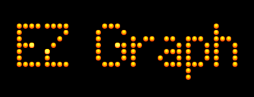# Conic Sections - The Hyperbola

On an (x, y) graph a hyperbola can have the shape shown to the left.Here's a link to EZ Graph - Hyperbola. This is a graphic calculator presenting functions that draw hyperbolas.

If a plane intersects the cone when it is parallel to the axis, the conic section is a hyperbola. This is shown to the left.Click this link for an interactive 3D animation showing how a cone and plane intersect to form a hyperbola.

Here is a video that shows several possibilities for forming a hyperbola by intersecting a cone and a plane.

Custom Search